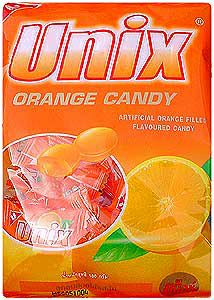# Manual Pages  — SQRT

### NAME

cbrt, cbrtf, cbrtl, sqrt, sqrtf, sqrtl – cube root and square root functions

### LIBRARY

Math Library (libm, -lm)

### SYNOPSIS

`#include <math.h>`

double
cbrt(double x);

float
cbrtf(float x);

long double
cbrtl(long double x);

double
sqrt(double x);

float
sqrtf(float x);

long double
sqrtl(long double x);

### DESCRIPTION

The cbrt(), cbrtf(), and cbrtl() functions compute the cube root of x.

The sqrt(), sqrtf(), and sqrtl() functions compute the non-negative square root of x.

### RETURN VALUES

The cbrt(), cbrtf(), and cbrtl() functions return the requested cube root. The sqrt(), sqrtf(), and sqrtl() functions return the requested square root unless an error occurs. An attempt to take the sqrt() of negative x raises an invalid exception and causes an NaN to be returned (except that the square root of -0 is valid and equal to -0.)

### STANDARDS

The cbrt(), cbrtf(), cbrtl(), sqrt(), sqrtf(), and sqrtl() functions conform to ISO/IEC 9899:1999 ("ISO C99").

### HISTORY

The cbrt() function appeared in BSD 4.3 . The sqrtl() function appeared in FreeBSD 8.0 . The cbrtl() function appeared in FreeBSD 9.0 .

 SQRT (3) March 5, 2011